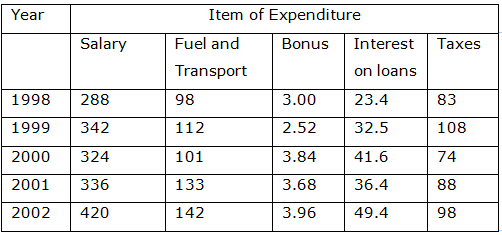# Direction (1 – 4): Study the following table and answer the questions based on it. Expenditures of a Company (in Lakh Rupees) per Annum Over the given Years1. What is the average amount of interest per year which the company had to pay during this period?

(a) Rs. 32.43 lakhs

(b) Rs. 33.72 lakhs

(c) Rs. 34.18 lakhs

(d) Rs. 36.66 lakhs

(e) None of these

(d) Rs. 36.66 lakhs

(d) Average amount of interest paid by the Company during the given period

= Rs. (23.4+32.5+41.6+36.4+49.4)/5 lakhs

= Rs. 183.3/5 lakhs

= Rs. 36.66 lakhs.

2. The total amount of bonus paid by the company during the given period is approximately what percent of the total amount of salary paid during this period?

(a) 0.1%

(b) 0.5%

(c) 1%

(d) 1.25%

(e) None of these

(c) 1%

(c) Required Percentage = (3.00+2.52+3.84+3.68+3.96)/(288+342+324+336+420)*100%

= 17/1710*100%

=1%

3. Total expenditure on all these items in 1998 was approximately what percent of the total expenditure in 2002?
(a) 62%

(b) 66%

(c) 69%

(d) 71%

(e) None of these

(c) 69%

Required percentage = (288+98+3.00+23.4+83)/(420+142+3.96+49.4+98)*100%

= 495.4/713.36*100*

=69.45%

4. The ratio between the total expenditure on Taxes for all the years and the total expenditure on Fuel and Transport for all the years respectively is approximately?

(a) 4:7

(b) 10:13

(c) 15:18

(d) 5:8

(e) None of these

(b) 10:13

Required ratio = (83+108+74+88+98)/(98+112+101+133+142)

=451/586

=1/1.3

=10/13

Directions (5 9 ): Study the table and answer the question that follows:

Data Related to Human Resource of a Multinational Company (X) which has 146 Offices across 8 Countries.

 Countries Officers Total no. employees Respective of male and Females employees Percentage of postgraduate employees A 16 2568 5:7 75 B 18 2880 11:5 65 C 14 2310 10:11 40 D 22 3575 3:2 60 E 13 2054 7:6 50 F 17 2788 20:21 75 G 24 3720 8:7 55 H 21 3360 9:5 80

5. If the number of male post-graduate employees in country H is 1800, what percent of female employees in that particular country is post-graduate?

(a) 76

(b) 74

(c) 72

(d) 64

(e) 68

(c) 72

n country H, 80% are post-graduate. That is = [80/100]*3360 = 2688
Male is given 1800. Hence, female post-graduate employees = 2688 – 1800 = 888
Total female employees is = [5/14]*3360 = 1200
Hence, required percentage = [888/1200]*100 = 74 Percent.

6. In which of the given countries is the percentage of women employees to the number of employees (both male and female) in that country the second lowest?

(a) G

(b) B

(c) E

(d) H

(e) D

(d) H Country B = 11:5 à [5/16]*100
Now, let’s say this is approximately 30% (16*3 = 48 which is close to 50).
Country D = [2/5]*100 = 40%
Country E = [6/13]*100 = approx. 45%
Country G = [7/15]*100 = approx. 45%
Country H = [5/14]*100 = approx. 35%
Now, second highest is Country H. Question solved.

7. What is the respective ratio between total number of male employees in countries B and H together and total number of female employees in countries C and D together?

(a) 63:52

(b) 51:38

(c) 77:64

(d) 69:44

(e) 57:40

(d) 69:44

Total male employees from countries B and H = [11/16]*2880 + [9/14]*3360 = 1980 + 2160 = 4140
Total female employees from Countries C and D = [11/21]*2310 + [2/5]*3575 = 1210 + 1430 = 2640
Required ratio = 4140:2640 = 69:44.

8. What is the difference between average number of post-graduate employees in countries A, B and D together and average number of post-graduate employees in countries F, G and H together?

(a) 282

(b) 276

(c) 316

(d) 342

(e) 294

(e) 294

(e) 75% of 2568 is required. Divide 2788 in four parts and add three parts. Thus 2568/4 is 642 and 642*3 = 1926
65% of 2880 is required. Divide 2880 in ten parts and add six parts and half of 7th part. Thus 288*6 + 288/2 = 1728 + 144 = 1872
60% of 3575 is required. Divide 3575 in 5 parts and add three parts. Thus 3575/5 = 715 and 715*3 = 2145
Average of these three is = [1/3]*[1926+1872+2145] = 1981
Using similar procedures, average of other three is calculated as = 2275
Difference = 2275 – 1981 = 294.

9. Which of the given countries has the highest number of average employees per office?

(a) F

(b) H

(c) B

(d) C

(e) D